Related Articles
ML | Adjusted R-Square in Regression Analysis
• Last Updated : 12 Jul, 2019

Prerequisite : Linear Regression, R-square in Regression

R-square test is used to determine the goodness of fit in regression analysis. Goodness of fit implies how better regression model is fitted to the data points. More is the value of r-square near to 1, better is the model. But the problem lies in the fact that the value of r-square always increases as new variables(attributes) are added to the model, no matter that the newly added attributes have a positive impact on the model or not. also, it can lead to over fitting of the model if there are large no. of variables.

Adjusted r-square is a modified form of r-square whose value increases if new predictors tend to improve model’s performance and decreases if new predictors does not improve performance as expected.

For better understanding consider :
Average Fitted Line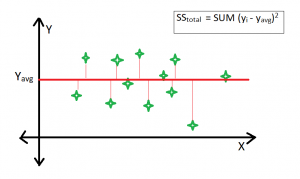Best Fitted Line :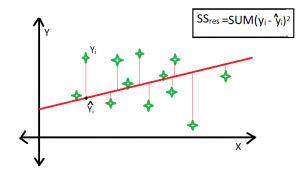R-square formula: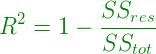Clearly, SStot is always fixed for some data points if new predictors are added to the model, but value of SSres decreases as model tries to find some correlations from the added predictors. Hence, r-square’s value always increases.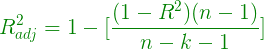Here, k is the no. of regressors and n is the sample size.
if the newly added variable is good enough to improve model’s performance, then it will overwhelm the decrease due to k. Otherwise, increase in k will decrease adjusted r-square value.

Example-

Case #1:
 `import` `pandas as pd``import` `numpy as np`` ` `# Download dataset and add complete path of the dataset.``# Importing dataset``s ``=` `pd.read_csv(``'Salary_Data.csv'``)`` ` ` ` `# Used to standarise statsmodel in python``f ``=` `np.ones((``30``, ``1``))``s.insert(``0``, ``'extra'``, f)`` ` `# Gives summary of data model->gives value of r-square and adjusted r-square``import` `statsmodels.formula.api as sm``X_opt ``=` `s.iloc[:, :``-``1``]``Y1 ``=` `s.iloc[:, ``-``1``]`` ` ` ` `regressor_OLS ``=` `sm.OLS(endog ``=` `Y1, exog ``=` `X_opt).fit()``regressor_OLS.summary()`

Output :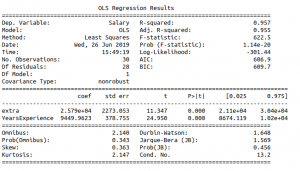Summary Table

Case #2:

 `import` `pandas as pd``import` `numpy as np`` ` `# Download dataset and add complete path of the dataset.``# Importing dataset``s ``=` `pd.read_csv(``'Salary_Data.csv'``)`` ` ` ` `# Used to standarise statsmodel in python``f ``=` `np.ones((``30``, ``1``))``s.insert(``0``, ``'extra'``, f)`` ` `# Inserting a new column to datset having employee ids``g ``=``[]``for` `i ``in` `range``(``1``, ``31``):``    ``g.append(i)`` ` `s.insert(``2``, ``'Id'``, g)`` ` ` ` `# Gives summary of data model->gives value of r-square and adjusted r-square``import` `statsmodels.formula.api as sm``X_opt ``=` `s.iloc[:, :``-``1``]``Y1 ``=` `s.iloc[:, ``-``1``]`` ` ` ` `regressor_OLS ``=` `sm.OLS(endog ``=` `Y1, exog ``=` `X_opt).fit()``regressor_OLS.summary()`

Output :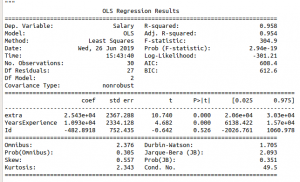Summary Table

Explanation –
R-square value and adjusted r-square value 0.957, 0.955 respectively. But when an attribute `Id` is added, which is an irrelevant attribute, gives r-square and adjusted r-square equal to
0.958, 0.954 respectively.
Hence on adding an irrelevant attribute in dataset, the value of r-square increases(from 0.957 to 0.958). But value of adjusted r-square decreases(from 0.955 to 0.954).My Personal Notes arrow_drop_up